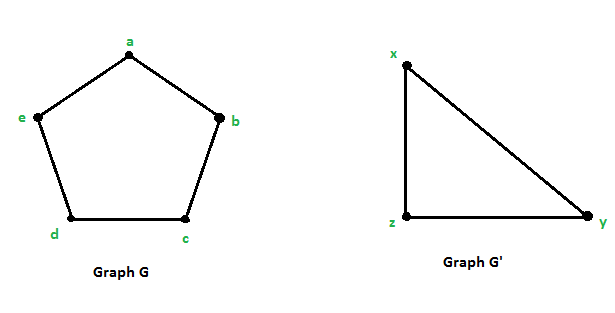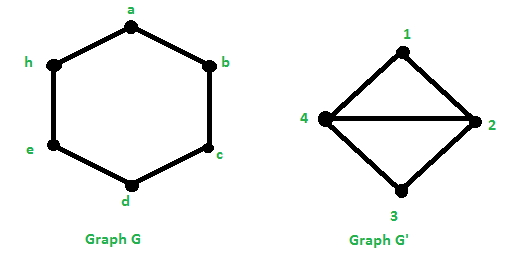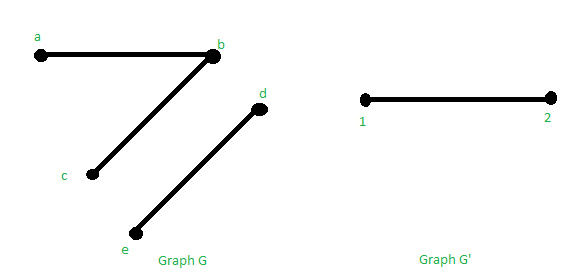Related Articles

# Graph Homomorphism

• Last Updated : 04 Jul, 2021

A graph G is a collection of a set of vertices and a set of edges that connects those vertices. It consists of two sets:

• Set of Vertices: V = {v1, v2, …, vn}
• Set of edges: E = {e1, e2, …, en}

The graph G is denoted as G = (V, E).

Homomorphism of Graphs: A graph Homomorphism is a mapping between two graphs that respects their structure, i.e., maps adjacent vertices of one graph to the adjacent vertices in the other. A homomorphism from graph G to graph H is a map from VG to VH which takes edges to edges.

Definition: A graph homomorphism F from a graph G = (V, E) to a graph G’ = (V’, E’) is written as:

f : G –> G’
It is a mapping f: V –> V’ from the vertex set of G to the vertex set of G’ such that {u, v} ∈ E ⇒ {f(u), f(v) ∈ E’

The above definition is extended to the directed graphs. Then, for a homomorphism f: G –> G’ is; {f(u), f(v)} is an arc of G’ only if (u, v) is an arc of G. If there exists a homomorphism; f: G –> G’, then it is written as G–>G’ G is said to be homomorphic to G’.

Isomorphism: If the homomorphism f: G –> G’ is a bijection (one-one and onto mapping) whose inverse is also a graph homomorphism, then f is a graph isomorphism.

Example 1: Below are the 2 graphs G = (V, E) with V = {a, b, c, d, e} and E = {(a, b), (b, c), (c, d), (d, e), (e, a)} and G’ = (V’, E’) with V’ = {x, y, z} and E’ = {(x, y), (y, z), (z, x)}.There exists a mapping f: G –> G’ such that {u, v} ∈ E ⇒ {f(u), f(v)} ∈ E’.

Solution:

Let us say that f(a) = x, f(b) = y, f(c) = z, f(d) = x and f(e) = z.

• If (a, b) is an edge in G, then (f(a), f(b)) must be an edge in E’.
`f(a) = x and f(b) = y  ⇒  (f(a), f(b)) = (x, y) ∈ E'`
• If (b, c) is an edge in G, then (f(b), f(c)) must be an edge in E’.
`f(b) = y and f(c) = z  ⇒  (f(b), f(c)) = (y, z) ∈ E'`
• If (c, d) is an edge in G, then (f(c), f(d)) must be an edge in E’.
`f(c) = z and f(d) = x  ⇒  (f(c), f(d)) = (z, x) ∈ E'`
• If (d, e) is an edge in G, then (f(d), f(e)) must be an edge in E’
`f(d) = x and f(e) = z  ⇒  (f(d), f(e)) = (x, z) ∈ E'`
• If (e, a) is an edge in G, then (f(e), f(a)) must be an edge in E’
`f(e) = z and f(a) = x  ⇒  (f(c), f(d)) = (z, x) ∈ E'`

Therefore, it can be seen that ∀{u, v} ∈ E ⇒ ∃{f(u), f(v)} ∈ E’. So f is a homomorphism.

Example 2: Below are the 2 graphs G = (V, E) with V = {a, b, c, d, e, h} and E = {(a, b), (b, c), (c, d), (d, e), (e, h), (f, a) } and G’ = (V’, E’) with V’ = {1, 2, 3, 4} and
E’ = { (1, 2), (2, 3), (3, 4), (4, 1), (4, 2)}.There exists a mapping : G–> G’ such that {u, v} ∈ E ⇒ {f(u), f(v)} ∈ E’.

Solution:

Let us say that f(a) = 1, f(b) = 4, f(c) = 2, f(d) = 4, f(e) = 2 and f(h) =  4.

• If (a, b) is an edge in G, then (f(a), f(b)) must be an edge in E’.
`f(a) = 1 and f(b) = 4  ⇒  (f(a), f(b)) = (1, 4) ∈ E'`
• If (b, c) is an edge in G, then (f(b), f(c)) must be an edge in E’.
`f(b) = 4 and f(c) = 2  ⇒  (f(b), f(c)) = (4, 2) ∈ E'`
• If (c, d) is an edge in G, then (f(c), f(d)) must be an edge in E’.
```f(c) = 2 and f(d) = 4  ⇒  (f(c), f(d)) = (2, 4) ∈ E'.
Note- (2, 4) is the same as (4, 2).```
• If (d, e) is an edge in G, then (f(d), f(e)) must be an edge in E’.
`f(d) = 4 and f(e) = 2  ⇒  (f(d), f(e)) = (4, 2) ∈ E'`
• If (e, h) is an edge in G, then (f(e), f(h)) must be an edge in E’.
`f(e) = 2 and f(a) = 4 ⇒  (f(c), f(d)) = (2, 4) ∈ E'`
• If (h, a) is an edge in G, then (f(h), f(a)) must be an edge in E’.
`f(h) = 4 and f(a) = 1 ⇒  (f(c), f(d)) = (4, 1) ∈ E'`

Therefore, it can be seen that ∀{u, v} ∈ E ⇒ ∃{f(u), f(v)} ∈ E’. So f is a homomorphism.

A homomorphism from a graph G to a graph H is a map from VG to VH which maps:

• Edges to Edges.
• Non-Edges to vertex, edge or non-edge.

Example 3: Below are the 2 graphs G = (V, E) with V = {a, b, c, d, e} and E = {(a, b), (b, c), (d, e), (e, h)} and G’ = (V’, E’) with V’ = {1, 2} and E’ = { (1, 2)}.Solution:

Let us say that f(a) = 1, f(b) = 2, f(c) = 1, f(d) = 2, f(e) = 1

• If (a, b) is an edge in G, then (f(a), f(b)) must be an edge in E’.
`f(a) = 1 and f(b) = 2 ⇒  (f(a), f(b)) = (1, 2) ∈ E'`
• If (b, c) is an edge in G, then (f(b), f(c)) must be an edge in E’.
`f(b) = 2 and f(c) = 1  ⇒  (f(b), f(c)) = (2, 1) ∈ E'`
• If (d, e) is an edge in G, then (f(d), f(e)) must be an edge in E’.
`f(d) = 2 and f(e) = 1  ⇒  (f(d), f(e)) = (2, 1) ∈ E'`

Here, it can be seen that (b, e) is not an edge in G, but (fb), f(e) ) = (2, 1) is an edge in the graph G’.

Attention reader! Don’t stop learning now. Get hold of all the important DSA concepts with the DSA Self Paced Course at a student-friendly price and become industry ready.  To complete your preparation from learning a language to DS Algo and many more,  please refer Complete Interview Preparation Course.

In case you wish to attend live classes with experts, please refer DSA Live Classes for Working Professionals and Competitive Programming Live for Students.

My Personal Notes arrow_drop_up